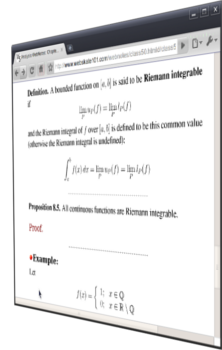Analysis WebNotes# Welcome to Analysis WebNotes

Analysis WebNotes is a self-contained course in Mathematical Analysis for undergraduates or beginning graduate students.

The site is organized into chapters and classes. There's also a set of homework exercises.

## Where do I start?

If you're searching for information on a particular area (say, uniform convergence) or a particular result (like the Mean Value Theorem), try the full chapter index or else the index of results.

If you're planning on using the site for a course, or to learn analysis on your own, then you may want to work through the sixty classes in the site in order.

## What is analysis?

Mathematical Analysis studies everything that involves limits. So it looks back at the material you learned in calculus, and explains why calculus works. At the same time, a course in analysis looks ahead to more advanced mathematics, such as topology, Fourier analysis, the theory of differential equations, complex analysis, etc.

Analysis is a proof-based subject, and so this site is full of theorems and their proofs. If you're interested in pure mathematics and want an introduction to proof-based mathematics, analysis is a great place to learn, because many of the results you see are close to things you've probably already seen in calculus.